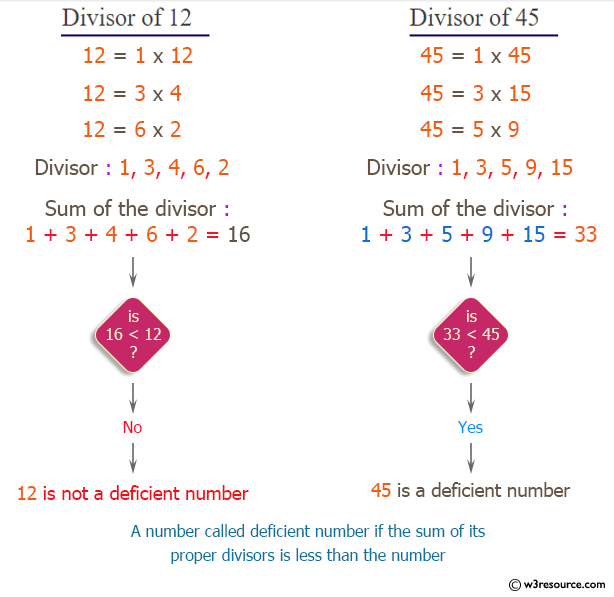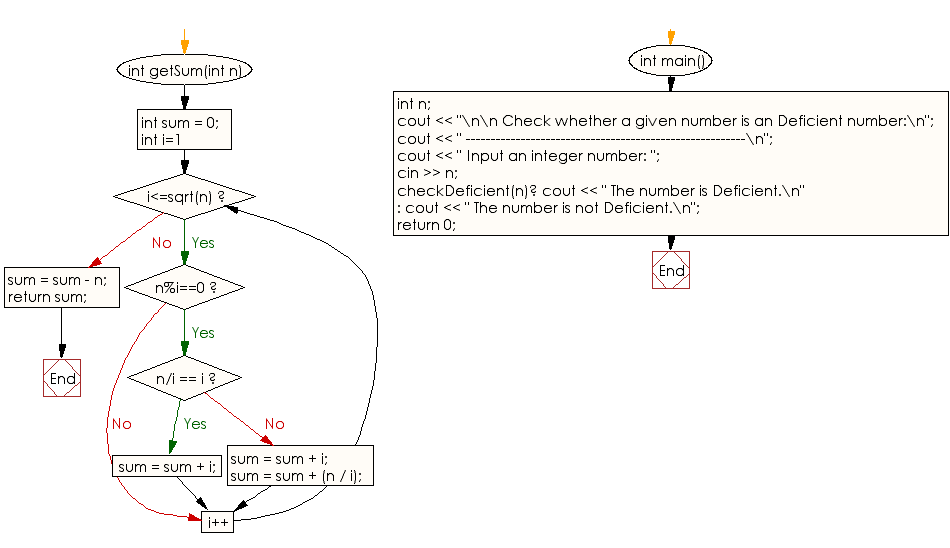﻿ C++ : Check whether a given number is Deficient or not

# C++ Exercises: Check whether a given number is Deficient or not

## C++ Numbers: Exercise-6 with Solution

Write a program in C++ to check whether a given number is Deficient or not.

Pictorial Presentation:Sample Solution:

C++ Code :

``````#include <bits/stdc++.h>
using namespace std;
int getSum(int n)
{
int sum = 0;
for (int i=1; i<=sqrt(n); i++)
{
if (n%i==0)
{
if (n/i == i)
sum = sum + i;
else
{
sum = sum + i;
sum = sum + (n / i);
}
}
}
sum = sum - n;
return sum;
}
bool checkDeficient(int n)
{
return (getSum(n) < n);
}
int main()
{
int n;
cout << "\n\n Check whether a given number is an Deficient number:\n";
cout << " --------------------------------------------------------\n";
cout << " Input an integer number: ";
cin >> n;
checkDeficient(n)? cout << " The number is Deficient.\n" : cout << " The number is not Deficient.\n";
return 0;
}
``````

Sample Output:

```Check whether a given number is an Deficient number:
--------------------------------------------------------
Input an integer number: 25
The number is Deficient.
```

Flowchart:C++ Code Editor: